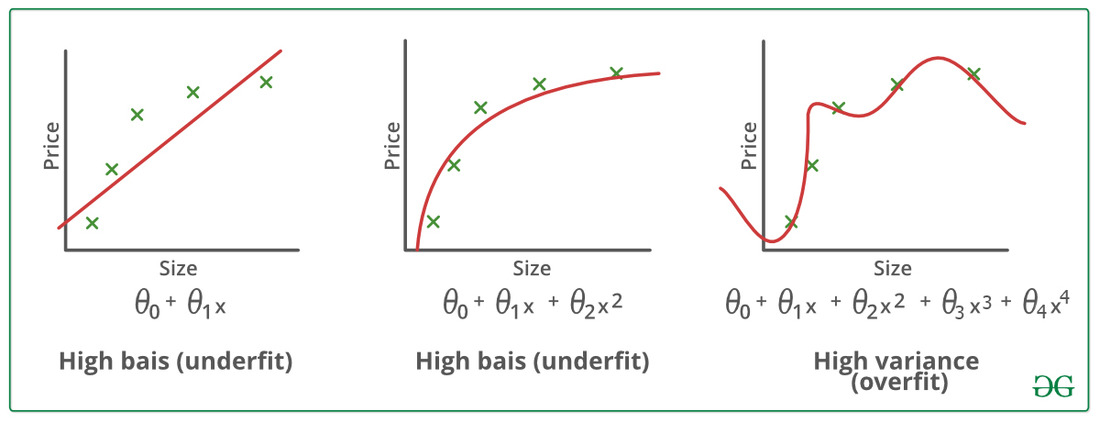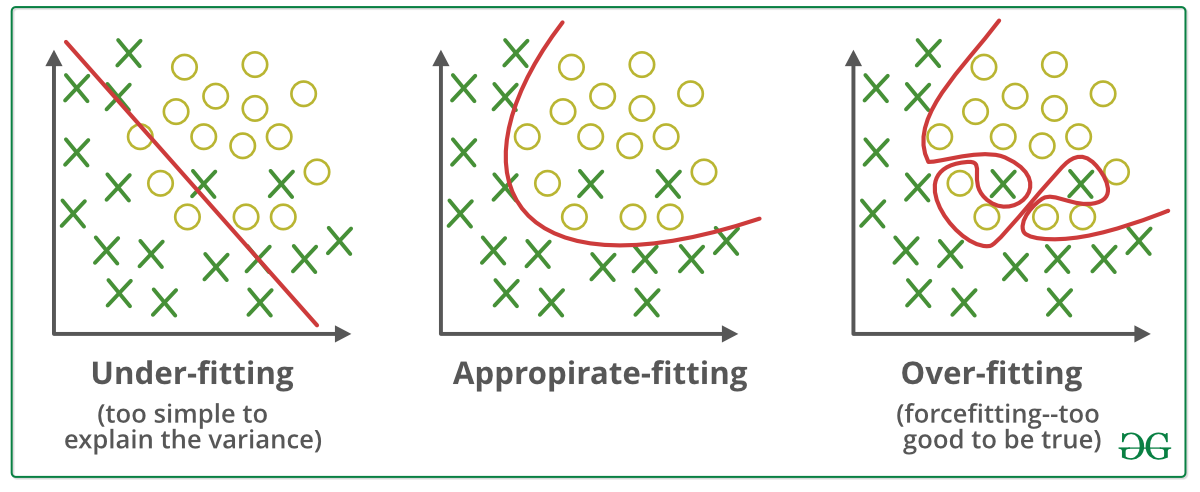# ML | Underfitting and Overfitting

• Difficulty Level : Medium
• Last Updated : 04 Aug, 2022

When we talk about the Machine Learning model, we actually talk about how well it performs and its accuracy which is known as prediction errors.  Let us consider that we are designing a machine learning model. A model is said to be a good machine learning model if it generalizes any new input data from the problem domain in a proper way. This helps us to make predictions about future data, that the data model has never seen. Now, suppose we want to check how well our machine learning model learns and generalizes to the new data. For that, we have overfitting and underfitting, which are majorly responsible for the poor performances of the machine learning algorithms.

Before diving further let’s understand two important terms:• Bias: Assumptions made by a model to make a function easier to learn. It is actually the error rate of the training data. When the error rate has a high value, we call it High Bias and when the error rate has a low value, we call it low Bias.
• Variance:  The difference between the error rate of training data and testing data is called variance. If the difference is high then it’s called high variance and when the difference of errors is low then it’s called low variance. Usually, we want to make a low variance for generalized our model.

Underfitting: A statistical model or a machine learning algorithm is said to have underfitting when it cannot capture the underlying trend of the data, i.e., it only performs well on training data but performs poorly on testing data. (It’s just like trying to fit undersized pants!) Underfitting destroys the accuracy of our machine learning model. Its occurrence simply means that our model or the algorithm does not fit the data well enough. It usually happens when we have fewer data to build an accurate model and also when we try to build a linear model with fewer non-linear data. In such cases, the rules of the machine learning model are too easy and flexible to be applied to such minimal data and therefore the model will probably make a lot of wrong predictions. Underfitting can be avoided by using more data and also reducing the features by feature selection.

In a nutshell, Underfitting refers to a model that can neither performs well on the training data nor generalize to new data.

Reasons for Underfitting:

1. High bias and low variance
2. The size of the training dataset used is not enough.
3. The model is too simple.
4. Training data is not cleaned and also contains noise in it.

Techniques to reduce underfitting:

1. Increase model complexity
2. Increase the number of features, performing feature engineering
3. Remove noise from the data.
4. Increase the number of epochs or increase the duration of training to get better results.

Overfitting: A statistical model is said to be overfitted when the model does not make accurate predictions on testing data. When a model gets trained with so much data, it starts learning from the noise and inaccurate data entries in our data set. And when testing with test data results in High variance. Then the model does not categorize the data correctly, because of too many details and noise. The causes of overfitting are the non-parametric and non-linear methods because these types of machine learning algorithms have more freedom in building the model based on the dataset and therefore they can really build unrealistic models. A solution to avoid overfitting is using a linear algorithm if we have linear data or using the parameters like the maximal depth if we are using decision trees.

In a nutshell, Overfitting is a problem where the evaluation of machine learning algorithms on training data is different from unseen data.

### Reasons for Overfitting are as follows:

1.  High variance and low bias
2. The model is too complex
3. The size of the training data

Examples:Techniques to reduce overfitting:

1. Increase training data.
2. Reduce model complexity.
3. Early stopping during the training phase (have an eye over the loss over the training period as soon as loss begins to increase stop training).
4. Ridge Regularization and Lasso Regularization
5. Use dropout for neural networks to tackle overfitting.

Good Fit in a Statistical Model: Ideally, the case when the model makes the predictions with 0 error, is said to have a good fit on the data. This situation is achievable at a spot between overfitting and underfitting. In order to understand it, we will have to look at the performance of our model with the passage of time, while it is learning from the training dataset.

With the passage of time, our model will keep on learning, and thus the error for the model on the training and testing data will keep on decreasing. If it will learn for too long, the model will become more prone to overfitting due to the presence of noise and less useful details. Hence the performance of our model will decrease. In order to get a good fit, we will stop at a point just before where the error starts increasing. At this point, the model is said to have good skills in training datasets as well as our unseen testing dataset.

My Personal Notes arrow_drop_up